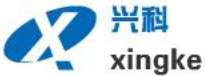##• 廊坊兴科化工材料有限公司
• 服务热线
18832653777
••• 产品中心

订购热线

18832653777

在线咨询

# 络合铁脱硫工艺

H2S + 1/2O2 → H2O + S （1）

H2S(g) + H2O(L)H2S(L) + H2O(L) （2）

（L）——液相，下同

H2S电离：

H2S(L)H+ (L)+ HS-(L) （3）

HS-(L)+ 2Fe3+(L) → 2Fe2+(L) + H+(L) + S↓ （4）

H2S(g) + 2Fe3+(L) → 2H+(L) + S↓+ 2Fe2+(L) （5）

1/2O2 (g) + H2O(L) → 1/2O2 (L) + H2O(L) （6）

1/2O2(L) + H2O(L) + 2Fe2+(L) → 2OH-(L) + 2Fe3+(L) （7）

1/2O2(g) + H2O(L) + 2Fe2+(L) → 2OH-(L) + 2Fe3+(L) （8）

2HS-(L) + 2O2(L) →S2O32-(L) + H2O(L) （9）

CO2 (g) + H2O (L) → H2CO3 (L) （10）

H2CO3 (L) → H+(L) + HCO3-(L) （11）

HCO3-(L) → CO32-(L) + H+ (L) （12）

CO2 (g) + H2O(L) → H2CO3(L) （13）

H2CO3(L) + 2KOH (L)→ K2CO3 (L)+ 2H2O(L) （14）

K2CO3(L) + H2CO3 (L)→ 2KHCO3(L) （15）

，而且循环液量小，装置尺寸小，并可直接生成单质硫，不存在二次污染问题。

http://www.lfxkcl.com

### 联系我们

•联系人：张春怀

•手机：18832653777

•地址：大城县北魏乡正村

•• 在线沟通，请点我在线咨询

•